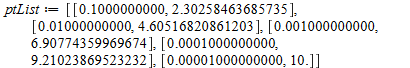# Question:dsolve, events: how to build an efficient loop

## Question:dsolve, events: how to build an efficient loop

Maple

My question is related to this discussion on "querying events"

http://www.mapleprimes.com/questions/125273-Dsolve-Events-How-To-Control-For-A-Sign-Change#comment125426

I thought I might start a new thread.

The following loop is an illustration of the sort of thing I'd like to do with dsolve and events: I loop over a parameter of the "event" and extract information on the solution at different values of the parameter. Here is the code (successfully tested on Maple 15 / Standard / Windows)

Slave :=
dsolve( {diff(y(t),t) = -y(t), y(0) = 1 }
, 'type' = numeric
, 'events' = [ [ y(t) = 10^(-p), 'halt' ] ]
, 'parameters' = [p]
) :
Master :=
subs( dummy = eval(Slave),
proc (P);
dummy('parameters' = ['p' = P]);
dummy;
end proc
) :

List := [ seq( Master(P)(10), P = 1..5) ] :
ptList := [ seq( [ 10^(-P), rhs(List[P,1]) ], P = 1..5 ) ];In words, I record the time t at which the event y(t)=10^(-p) triggers a halt, and then build a list of the (10^(-p),t) pairs. The idea here is to see how long it gets to approach ever more closely y(t)=0.

I have set up the system as a Slave/Master combination, following a suggestion by acer.

http://www.mapleprimes.com/posts/119858-Faster-Than-DEplot

But my loop is not terribly efficient because it keeps recalculating the solution from the beginning. It would be better to register an event without triggering a halt. I don't yet understand how to do that, despite Allan's very helpful suggestions (see the original discussion referenced right at the top)

I tried something like this:

Slave :=
dsolve( { diff(y(t),t) = -y(t), y(0) = 1, s(0) = 0 }
, 'type' = numeric
, 'discrete_variables' = [s(t)]
, 'events' = [ [ y(t) = 10^(-p), [ s(t) = s(t)+1 ] ] ]
, 'parameters' = [p]
) :

which says that when y(t) = 10^(-p) is satisfied, the discrete variable s(t) is incremented by 1. But it doesn't achieve what I intend. I think maybe the way to do this is to specify the initial condition like this y(0) = 10^(-p). I'm avoiding this approach because I'm looking for a method that would work on other problems where such initial conditions wouldn't be easy to rewrite.

I suspect there may be a simple way to write an efficient version of my loop above, one that does not set off a 'halt'. Any suggestions welcome! Thanks.

Edit. I didn't pay attention to the output. I should have run the loop for longer than 10 periods, since the next to last iteration almost needs 10 periods for the event to trigger. The very last item in the list is uninformative. So the 10 above should be replaced by 100, say.

Edit 2. Fixed a url.﻿